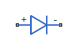# Diode

Piecewise linear diode in electrical systems

## Library

Electrical Elements

•## Description

The Diode block models a piecewise linear diode. If the voltage across the diode is bigger than the Forward voltage parameter value, then the diode behaves like a linear resistor with low resistance, given by the On resistance parameter value, plus a series voltage source. If the voltage across the diode is less than the forward voltage, then the diode behaves like a linear resistor with low conductance given by the Off conductance parameter value.

When forward biased, the series voltage source is described with the following equation:

`$V=Vf\left(1-{R}_{on}{G}_{off}\right),$`

where

 `V` Voltage `Vf` Forward voltage `Ron` On resistance `Goff` Off conductance

The Ron.Goff term ensures that the diode current is exactly zero when the voltage across it is zero. The reverse behavior is given byi/Goff, which is also zero at zero current.

### Variables

To set the priority and initial target values for the block variables prior to simulation, use the Initial Targets section in the block dialog box or Property Inspector. For more information, see Set Priority and Initial Target for Block Variables.

Nominal values provide a way to specify the expected magnitude of a variable in a model. Using system scaling based on nominal values increases the simulation robustness. Nominal values can come from different sources, one of which is the Nominal Values section in the block dialog box or Property Inspector. For more information, see Modify Nominal Values for a Block Variable.

## Parameters

Forward voltage

Minimum voltage that needs to be applied for the diode to become forward-biased. The default value is `0.6` V.

On resistance

The resistance of a forward-biased diode. The default value is `0.3` Ω.

Off conductance

The conductance of a reverse-biased diode. The default value is `1e-8` 1/Ω.

## Ports

The block has the following ports:

`+`

Electrical conserving port associated with the diode positive terminal.

`-`

Electrical conserving port associated with the diode negative terminal.

## Version History

Introduced in R2007a